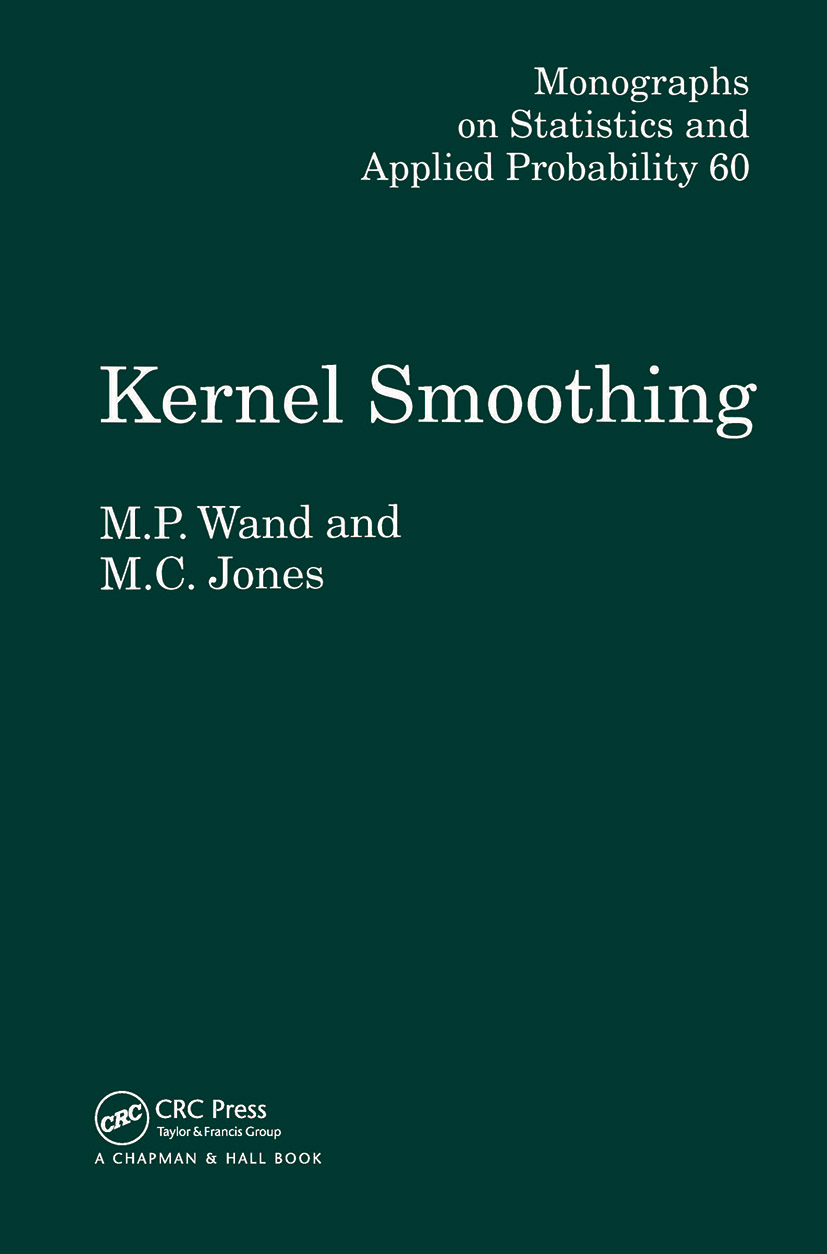# Kernel Smoothing

## 1st Edition

Chapman and Hall/CRC

224 pages

Hardback: 9780412552700
pub: 1994-12-01
SAVE ~\$37.00
\$185.00
\$148.00
x
eBook (VitalSource) : 9780429170591
pub: 1994-12-01
from \$111.00

FREE Standard Shipping!

### Description

Kernel smoothing refers to a general methodology for recovery of underlying structure in data sets.The basic principle is that local averaging or smoothing is performed with respect to a kernel function.

This book provides uninitiated readers with a feeling for the principles, applications, and analysis of kernel smoothers. This is facilitated by the authors' focus on the simplest settings, namely density estimation and nonparametric regression. They pay particular attention to the problem of choosing the smoothing parameter of a kernel smoother, and also treat the multivariate case in detail.

Kernel Smoothing is self-contained and assumes only a basic knowledge of statistics, calculus, and matrix algebra. It is an invaluable introduction to the main ideas of kernel estimation for students and researchers from other discipline and provides a comprehensive reference for those familiar with the topic.

More information on the book, and the accompanying R package can be found here.

Preface

Introduction

Introduction

Density estimation and histograms

Bibliographical notes

Univariate kernel density estimation

Introduction

The univariate kernel density estimator

The MSE and MISE criteria

Order and asymptotic notation; Taylor expansion

Order and asymptotic notation

Taylor expansion

Asymptotic MSE and MISE approximations

Exact MISE calculations

Canonical kernels and optimal kernel theory

Higher-older kernels

Measuring how difficult a density is to estimate

Modifications of the kernel density estimations

Local kernel density estimators

Variable kernel density estimators

Transformation kernel density estimators

Density estimation at boundaries

Density derivative estimation

Bibliographical notes

Exercises

Bandwidth selection

Introduction

Quick and simple bandwidth selectors

Normal scale rules

Oversmoothed bandwidth selection rules

Least squares cross-validation

Biased cross-validation

Estimation of density functionals

Plug-in bandwidth selection

Direct plug in rules

Solve-the-equation rules

Smoothed cross-validation bandwidth selection

Comparison of bandwidth selection

Theoretical performance

Bibliographical notes

Exercises

Multivariate kernel density estimation

Introduction

The multivariate kernel density estimator

Asymptotic MISE approximations

Exact MISE calculations

Choice of multivariate kernel

Choice of smoothing parametrisation

Bandwidth selection

Bibliographical notes

Exercises

Kernel regression

Introduction

Local polynomial kernel estimators

Asymptotic MSE approximations: linear case

Fixed equally spaced design

Random design

Asymptotic MSE approximations: general case

Behaviour near the boundary

Comparison with other kernel estimators

Asymptotic comparison

Effective kernels

Derivative estimation

Bandwidth selection

Multivariate nonparametric regression

Bibliographical notes

Exercises

Selected extra topics

Introduction

Kernel density estimation in other settings

Dependent data

Length biased data

Right-censored data

Data measured with error

Hazard function estimation

Spectral density estimation

Likelihood-based regression models

Intensity function estimation

Bibliographical notes

Exercises

Appendixes

A Notation

B Tables

C.1 Univariate normal densities

C.2 Multivariate normal densities

C.3 Bibliographical notes

D Computation of kernel estimators

D.1 Introduction

D.2 The binned kernel density estimator

D.3 Computation of kernel functional estimates

D.4 Computation of kernel regression estimates

D.5 Extension to multivariate kernel smoothing

D.6 Computing practicalities

D.7 Bibliographical notes

References

Index